Next: Gaussian kernel correlation integral Up: Correlation dimension Previous: Correlation dimension

### Takens-Theiler estimator

Convergence to a finite correlation dimension can be checked by plotting scale dependent ``effective dimensions'' versus length scale for various embeddings. The easiest way to proceed is to compute (numerically) the derivative of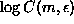with respect to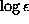, for example by fitting straight lines to the log-log plot of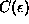. In Fig.(a) we see the output of the routine c2 acting on data from the NMR laser, processed by c2d in order to obtain local slopes. By default, straight lines are fitted over one octave in, larger ranges give smoother results. We can see that on the large scales, self-similarity is broken due to the finite extension of the attractor, and on small but yet statistically significant scales we see the embedding dimension instead of a saturated, m-independent value. This is the effect of noise, which is infinite dimensional, and thus fills a volume in every embedding space. Only on the intermediate scales we see the desired plateau where the results are in good approximation independent of m and. The region where scaling is established, not just the range selected for straight line fitting, is called the scaling range.

Since the statistical fluctuations in plots like Fig.(a) show characteristic (anti-)correlations, it has been suggested [78, 79] to apply a maximum likelihood estimator to obtain optimal values for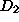. The Takens-Theiler-estimator reads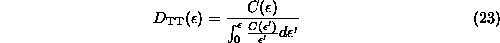and can be obtained by processing the output of c2 by c2t. Sinceis available only at discrete values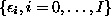, we interpolate it by a pure power law (or, equivalently, the log-log plot by straight lines: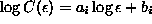) in between these. The resulting integrals can be solved trivially and summed:Plotting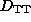versus(Fig.(b)) is an interesting alternative to the usual local slopes plot, Fig.(a). It is tempting to use such an ``estimator of dimension'' as a black box to provide a number one might quote as a dimension. This would imply the unjustified assumption that all deviations from exact scaling behavior is due to the statistical fluctuations. Instead, one still has to verify the existence of a scaling regime. Only then,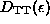evaluated at the upper end of the scaling range is a reasonable dimension estimator.Next: Gaussian kernel correlation integral Up: Correlation dimension Previous: Correlation dimension

Thomas Schreiber
Wed Jan 6 15:38:27 CET 1999